# The line x = a is a vertical asymptote of the curve y = f ( x ) .### Single Variable Calculus: Concepts...

4th Edition
James Stewart
Publisher: Cengage Learning
ISBN: 9781337687805### Single Variable Calculus: Concepts...

4th Edition
James Stewart
Publisher: Cengage Learning
ISBN: 9781337687805

#### Solutions

Chapter 2, Problem 5RCC

(a)

To determine

## To Describe: The line x=a is a vertical asymptote of the curve y=f(x).

Expert Solution

### Explanation of Solution

The vertical asymptote of the function y=f(x) is x=a, if y approaches ± as x approaches a from the right or left.

Limit is defined as limxaf(x)=± (or) limxa+f(x)=± (or) both

Graph: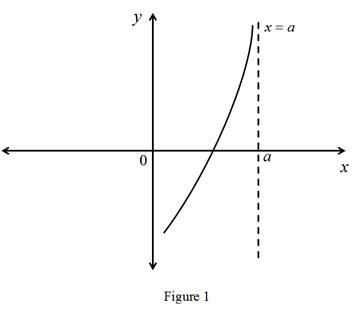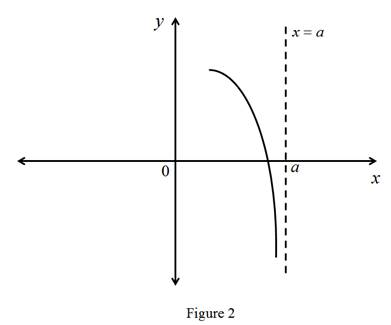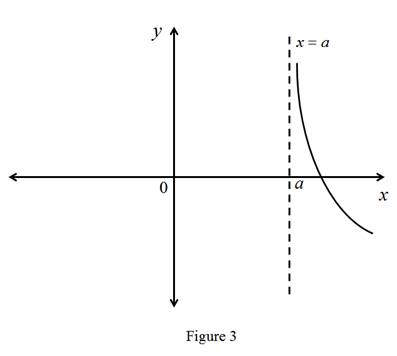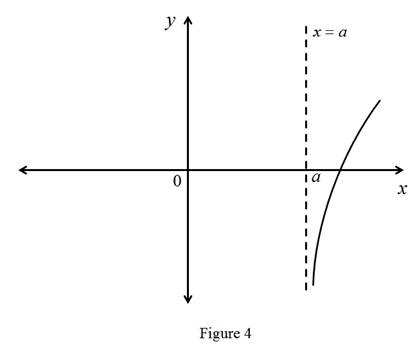(b)

To determine

Expert Solution

### Explanation of Solution

The horizontal asymptote of the function y=f(x) is y=b, if y approaches b as x approaches to ±.

Limit is defined as limxf(x)=b (or) limx+f(x)=b(or) both

Graph: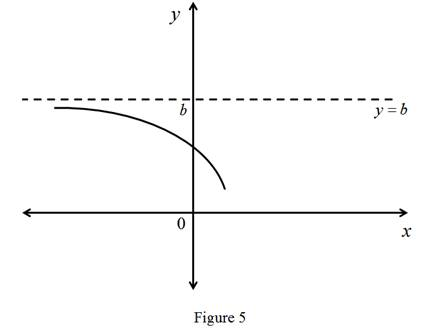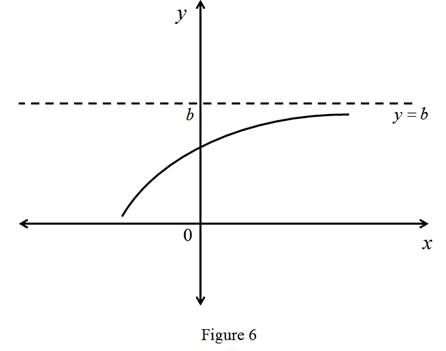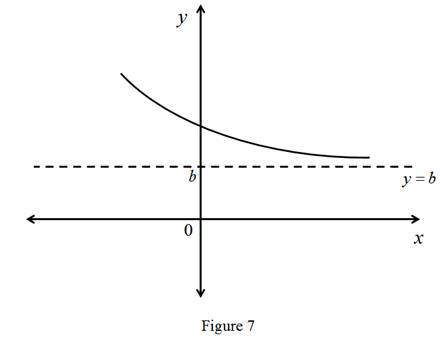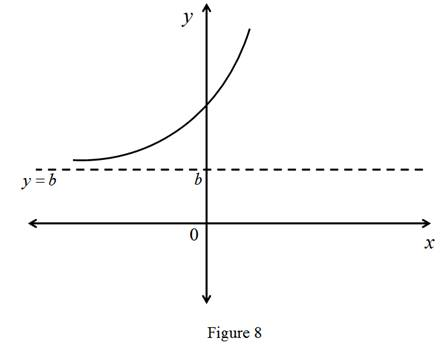### Have a homework question?

Subscribe to bartleby learn! Ask subject matter experts 30 homework questions each month. Plus, you’ll have access to millions of step-by-step textbook answers!